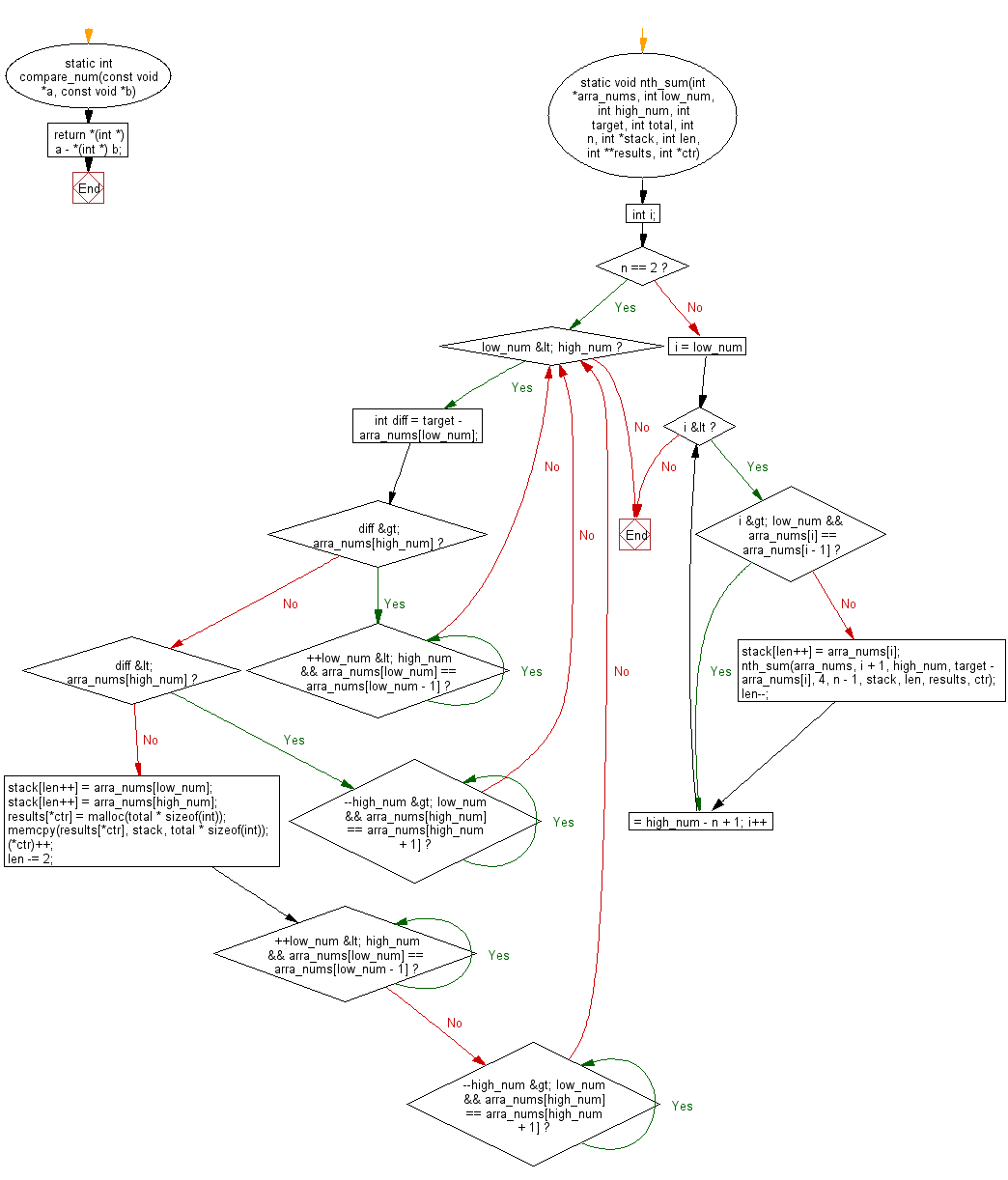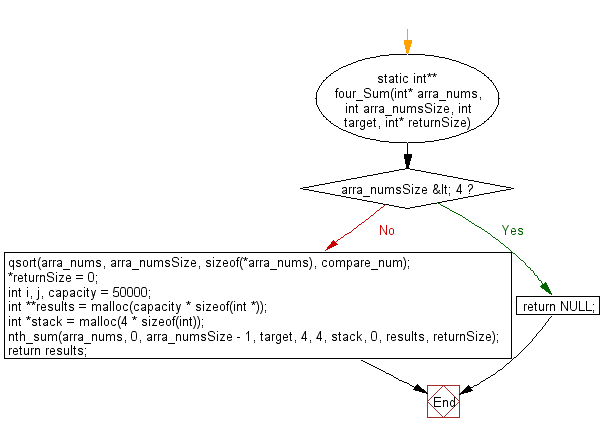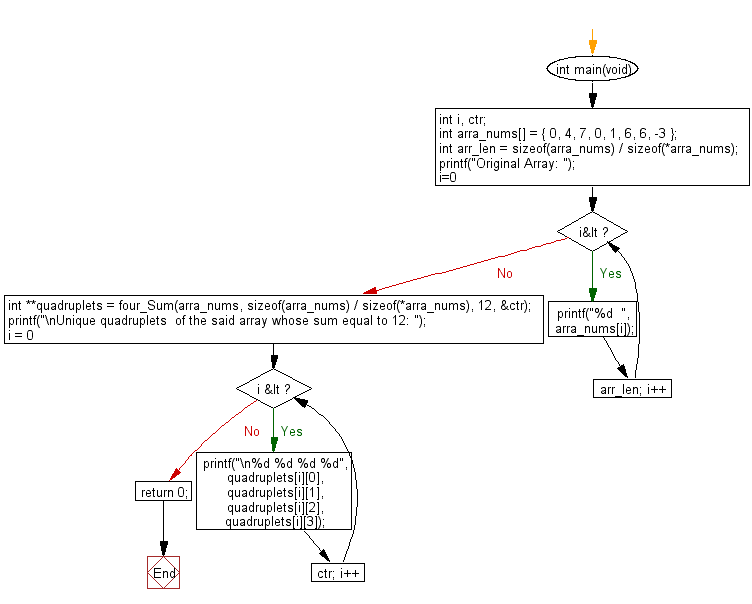﻿ C Program: All quadruplets in an array with zero sum# C Exercises: Find all unique quadruplets in a given array of integers whose sum equal to zero

## C Programming Practice: Exercise-9 with Solution

Write a C programming to find all unique quadruplets in a given array of integers whose sum equal to zero.

C Code:

``````#include <stdio.h>
#include <stdlib.h>
#include <string.h>

static int compare_num(const void *a, const void *b)
{
return *(int *) a - *(int *) b;
}

static void nth_sum(int *arra_nums, int low_num, int high_num, int target, int total,
int n, int *stack, int len, int **results, int *ctr)
{
int i;
if (n == 2) {
while (low_num < high_num) {
int diff = target - arra_nums[low_num];
if (diff > arra_nums[high_num]) {
while (++low_num < high_num && arra_nums[low_num] == arra_nums[low_num - 1]) {}
} else if (diff < arra_nums[high_num]) {
while (--high_num > low_num && arra_nums[high_num] == arra_nums[high_num + 1]) {}
} else {
stack[len++] = arra_nums[low_num];
stack[len++] = arra_nums[high_num];
results[*ctr] = malloc(total * sizeof(int));
memcpy(results[*ctr], stack, total * sizeof(int));
(*ctr)++;
len -= 2;
while (++low_num < high_num && arra_nums[low_num] == arra_nums[low_num - 1]) {}
while (--high_num > low_num && arra_nums[high_num] == arra_nums[high_num + 1]) {}
}
}
} else {
for (i = low_num; i <= high_num - n + 1; i++) {
if (i > low_num && arra_nums[i] == arra_nums[i - 1]) continue;
stack[len++] = arra_nums[i];
nth_sum(arra_nums, i + 1, high_num, target - arra_nums[i], 4, n - 1, stack, len,  results, ctr);
len--;
}
}
}

static int** four_Sum(int* arra_nums, int arra_numsSize, int target, int* returnSize)
{
if (arra_numsSize < 4) {
return NULL;
}

qsort(arra_nums, arra_numsSize, sizeof(*arra_nums), compare_num);

*returnSize = 0;
int i, j, capacity = 50000;
int **results = malloc(capacity * sizeof(int *));
int *stack = malloc(4 * sizeof(int));
nth_sum(arra_nums, 0, arra_numsSize - 1, target, 4, 4, stack, 0, results, returnSize);
return results;
}

int main(void)
{
int i, ctr;
int arra_nums[] = { 0, 4, 7, 0, 1, 6, 6, -3 };
int arr_len =  sizeof(arra_nums) / sizeof(*arra_nums);
printf("Original Array: ");
for(i=0; i<arr_len; i++)
{
printf("%d  ", arra_nums[i]);
}
int **quadruplets = four_Sum(arra_nums, sizeof(arra_nums) / sizeof(*arra_nums), 12, &ctr);
printf("\nUnique quadruplets  of the said array whose sum equal to 12: ");
for (i = 0; i < ctr; i++) {
}

return 0;
}
``````

Sample Output:

```Original Array: 0  4  7  0  1  6  6  -3
Unique quadruplets  of the said array whose sum equal to 12:
0 0 6 6
0 1 4 7
```

Flowchart: 1Flowchart: 2Flowchart: 3## C Programming Code Editor:

What is the difficulty level of this exercise?

Test your Programming skills with w3resource's quiz.

﻿

## C Programming: Tips of the Day

What is the difference between printf() and puts() in C?

puts is simpler than printf but be aware that the former automatically appends a newline. If that's not what you want, you can fputs your string to stdout or use printf.

Ref :https://bit.ly/3f2fwcH